# 数据挖掘从入门到放弃（一）：线性回归和逻辑回归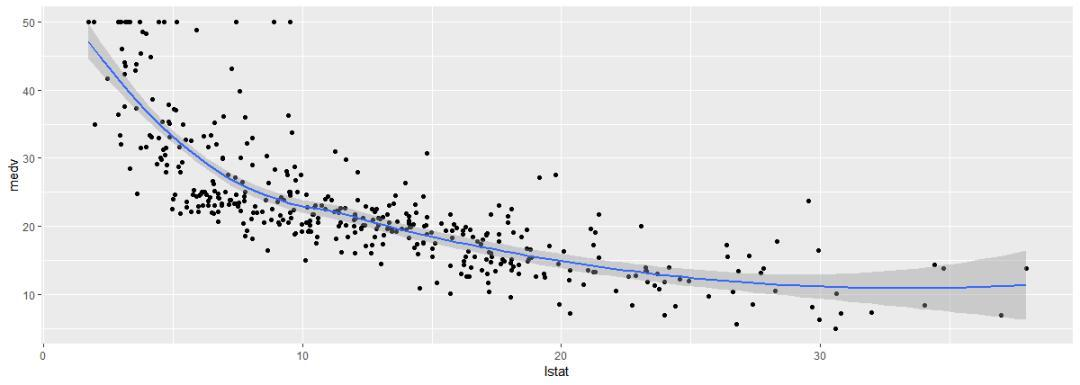## 一、理解线性回归模型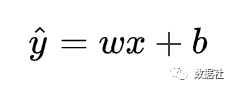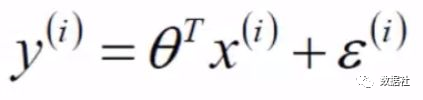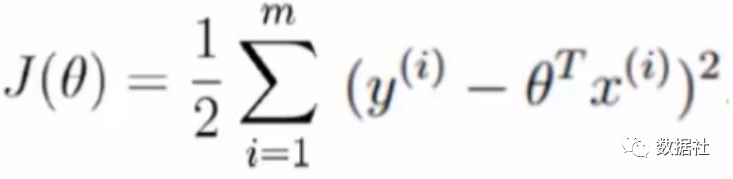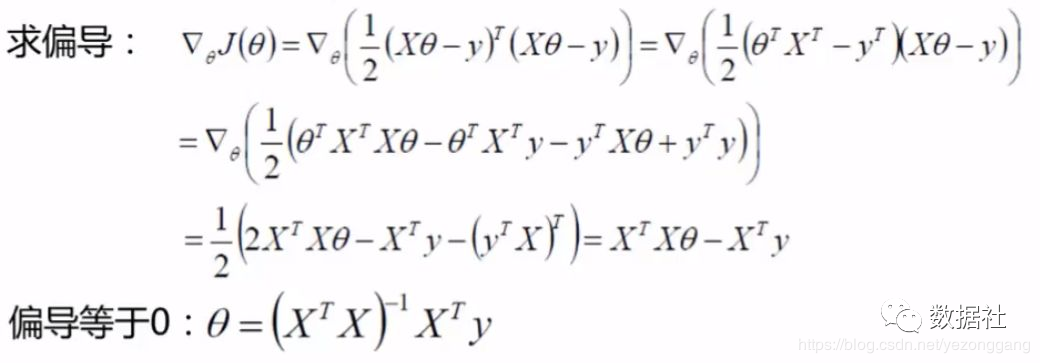## 二、计算机的做法：梯度下降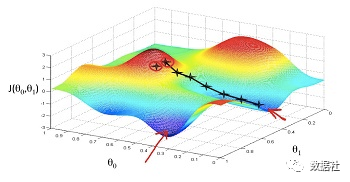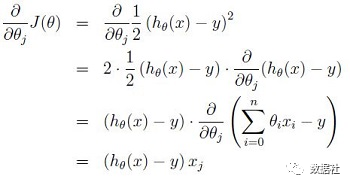θ 的每个分量更新公式为：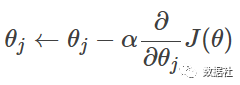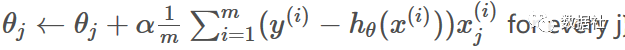## 三、逻辑回归模型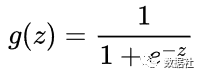sigmoid 函数图像是一个 S 曲线，取值在[0, 1]之间，在远离 0 的地方函数的值会很快接近 0 或者 1，sigmoid 函数的求导特性是：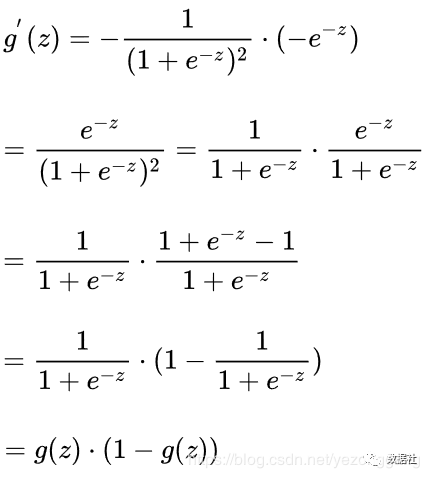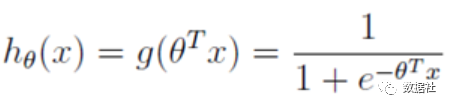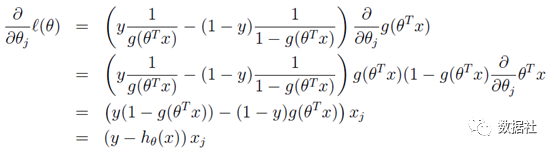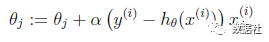## 四、回归模型使用

import pandas as pdfrom pandas import DataFrameimport numpy as npimport matplotlib.pyplot as plt%matplotlib inlineimport seaborn as snsfrom sklearn.linear_model import LogisticRegressionfrom sklearn.ensemble import RandomForestClassifierfrom sklearn.linear_model import LinearRegression# 数据读取baseUrl="C:\\Users\\71781\\Desktop\\2020\\ML-20200422\\houre_price\\"house_df=pd.read_csv(baseUrl+'train.csv' )test_df=pd.read_csv(baseUrl+'test.csv')house_df.head()# 删除无关变量house_df=house_df.drop(['saleTime','year','repairYear','latitude','longitude','buildingSize'],axis=1)test_df=test_df.drop(['saleTime','year','repairYear','latitude','longitude','buildingSize'],axis=1)# 模型建立X_price=house_df.drop(['price'],axis=1)# X_price.head()Y_price=house_df['price']Y_price.head()LR_reg=LinearRegression()LR_reg.fit(X_price, Y_price)Y_pred = LR_reg.predict(test_df)LR_reg.score(X_price, Y_price)# 可以选择进行特征缩放#new_house=house_df.drop(['price'],axis=1)#from sklearn.preprocessing import MinMaxScaler#minmax_scaler=MinMaxScaler().fit(new_house)   #进行内部拟合，内部参数会发生变化#scaler_housing=pd.DataFrame(minmax_scaler.transform(new_house),columns=new_house.columns)#mm=MinMaxScaler()#mm.fit(test_df)#scaler_t=mm.transform(test_df)#scaler_t=pd.DataFrame(scaler_t,columns=test_df.columns)## 评论